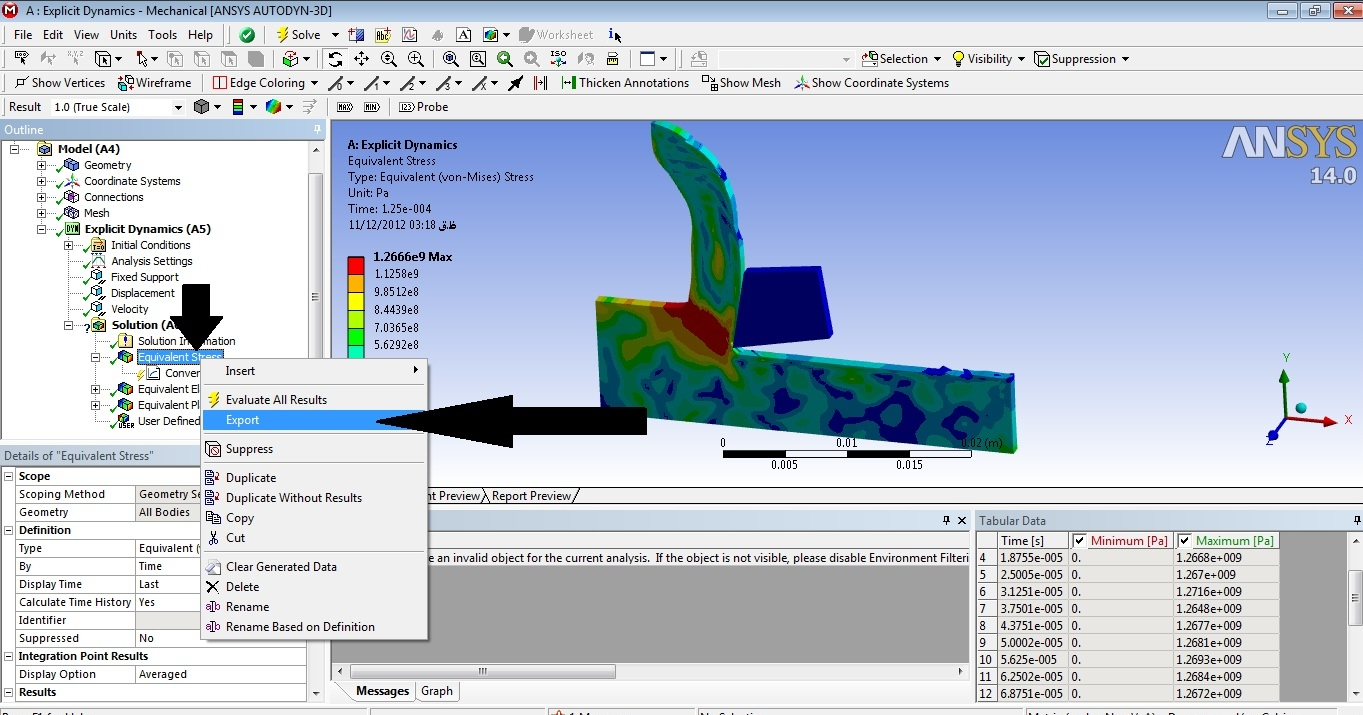tutorial 6 ( ansys workbench ) / ( cutting tools simulation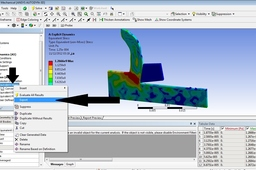just be aware of that the question input as if ( speed , time , dimension ) is not real it mean you can't use it as academic project . i just wanted to show you the processing of cutting tools simulation therefore with alter input data you can use it as real project .

for real input data you can use
cutting tools simulation

1. Step 1: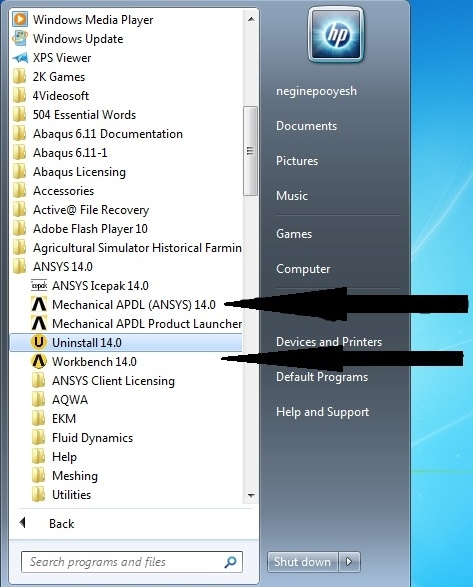2. Step 2:

choose explicit dynamic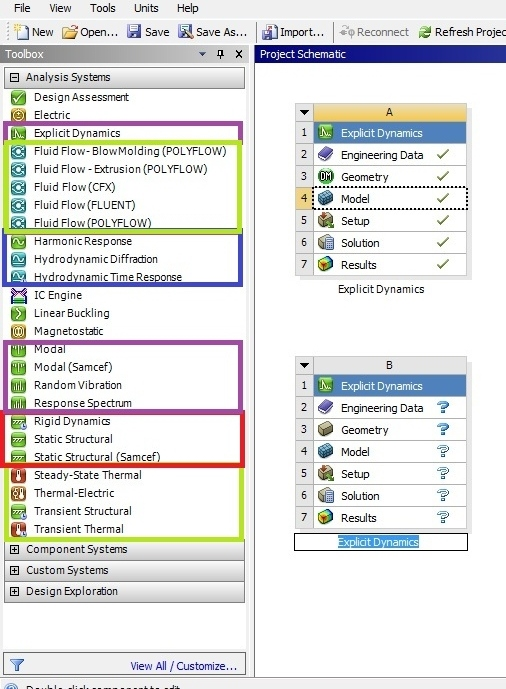3. Step 3: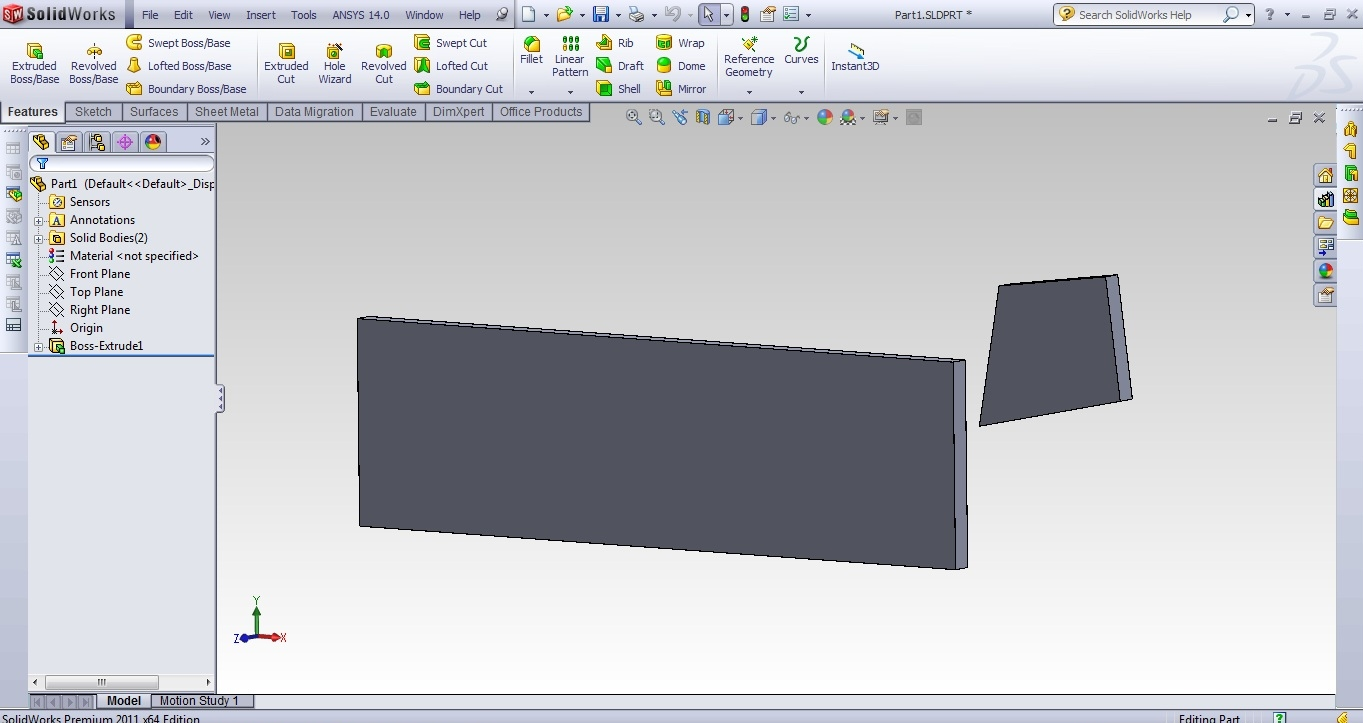4. Step 4: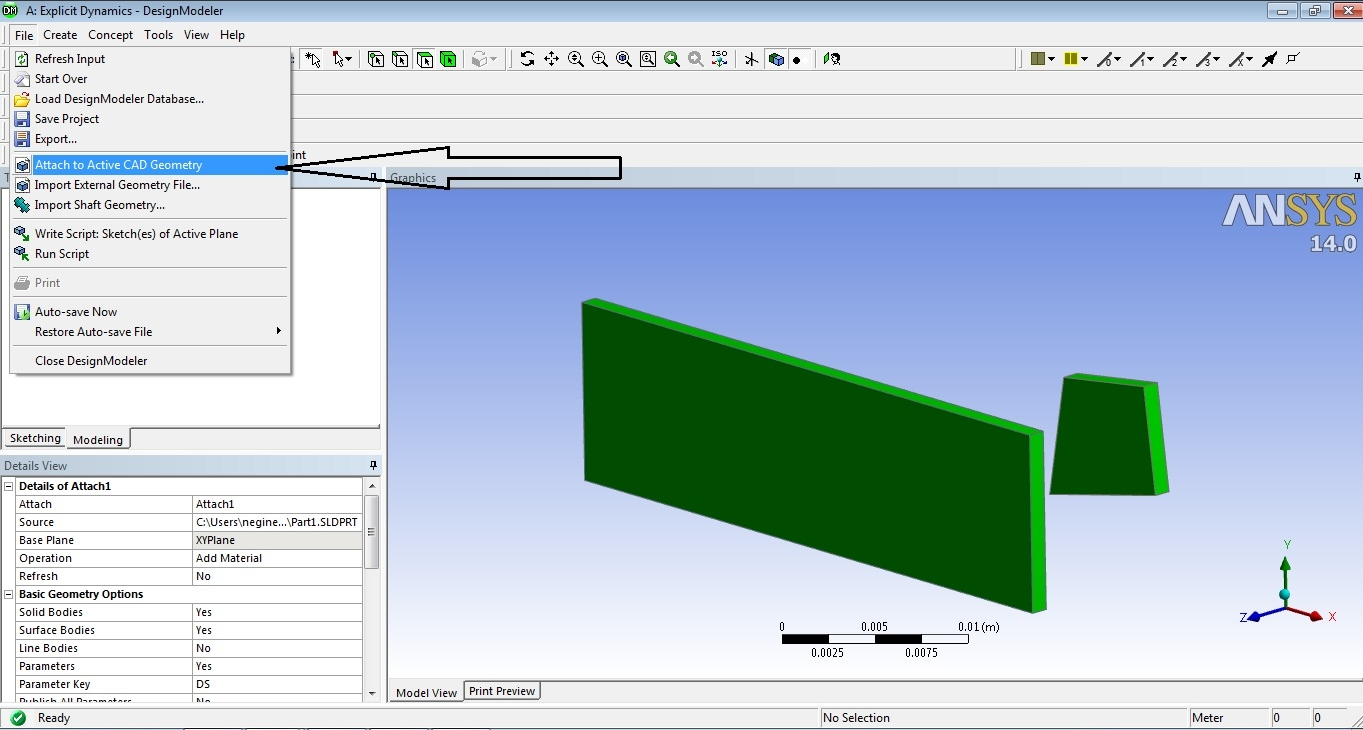5. Step 5: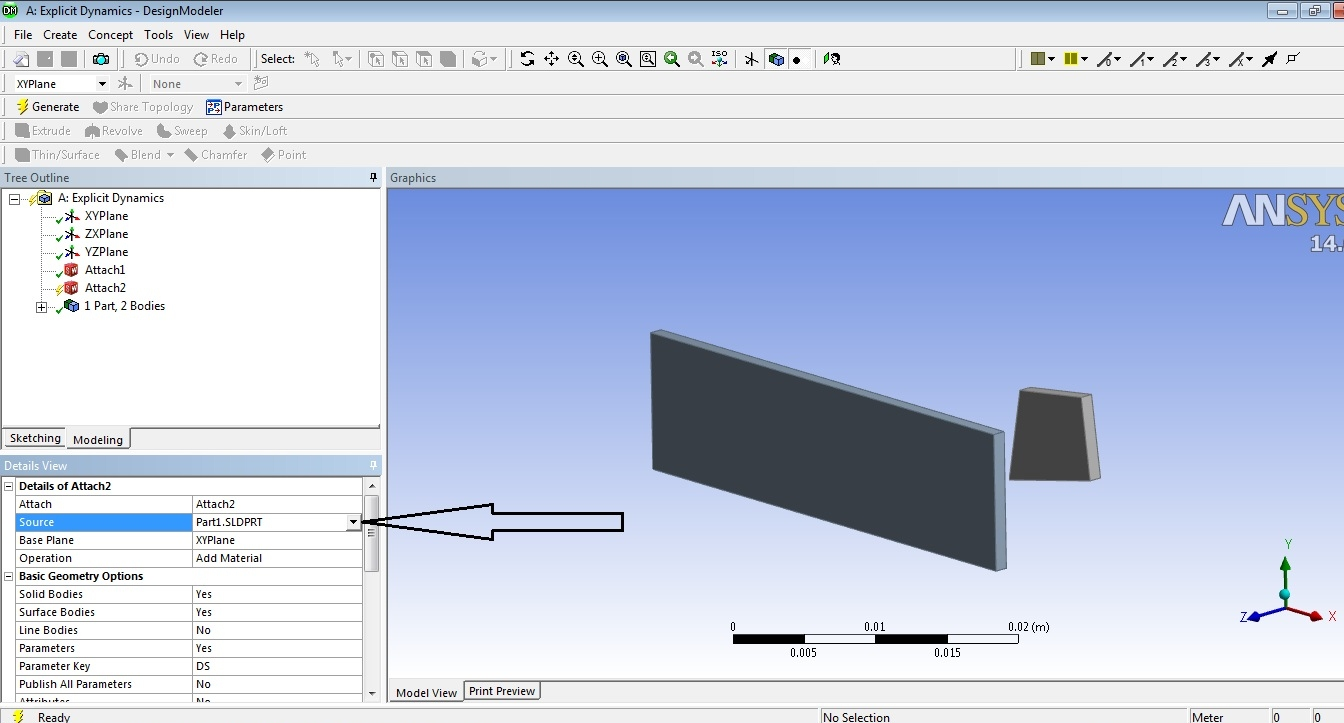6. Step 6: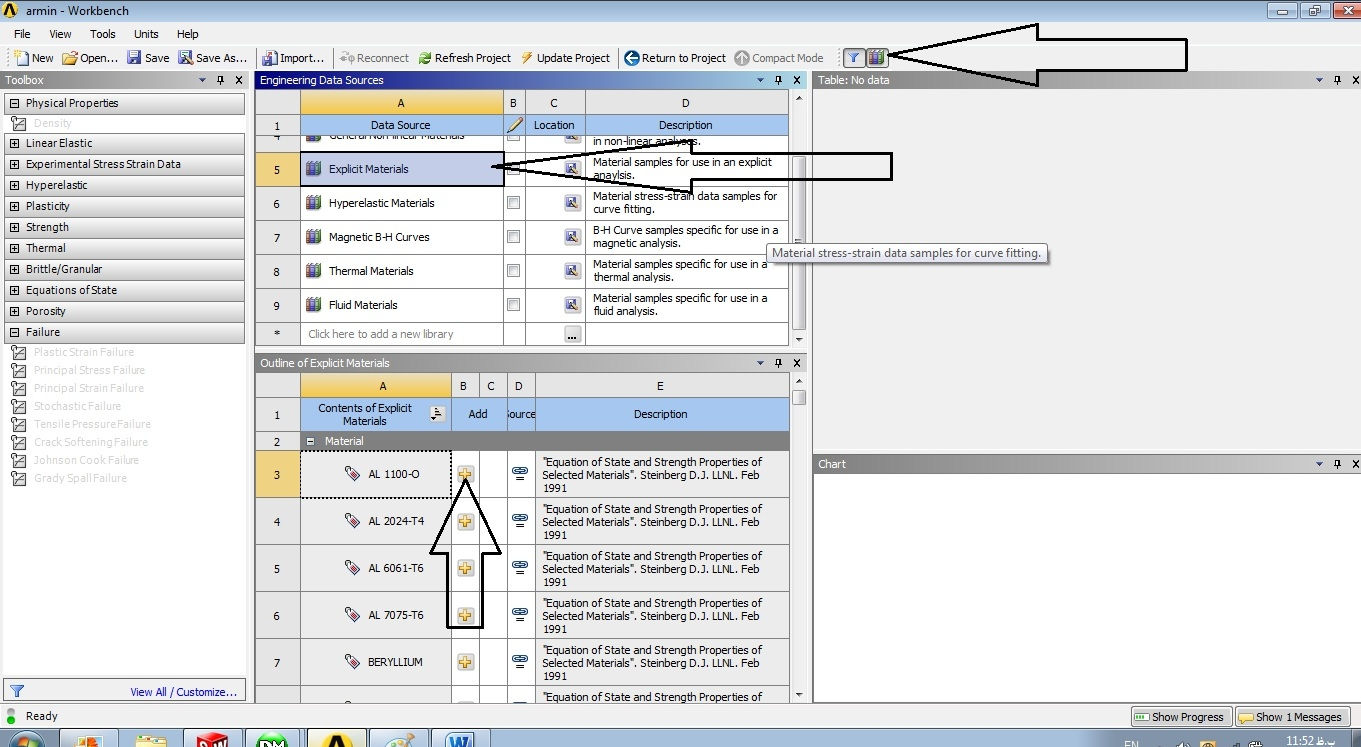7. Step 7: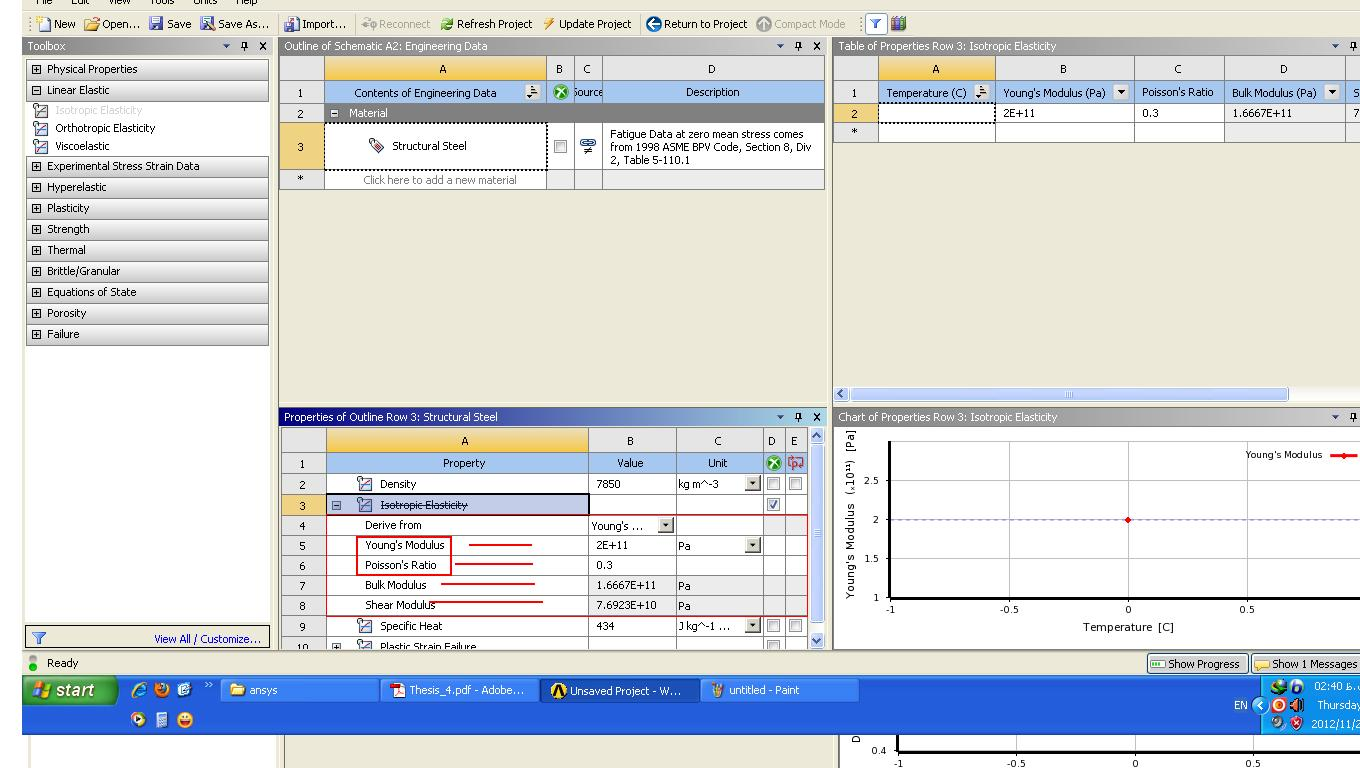8. Step 8: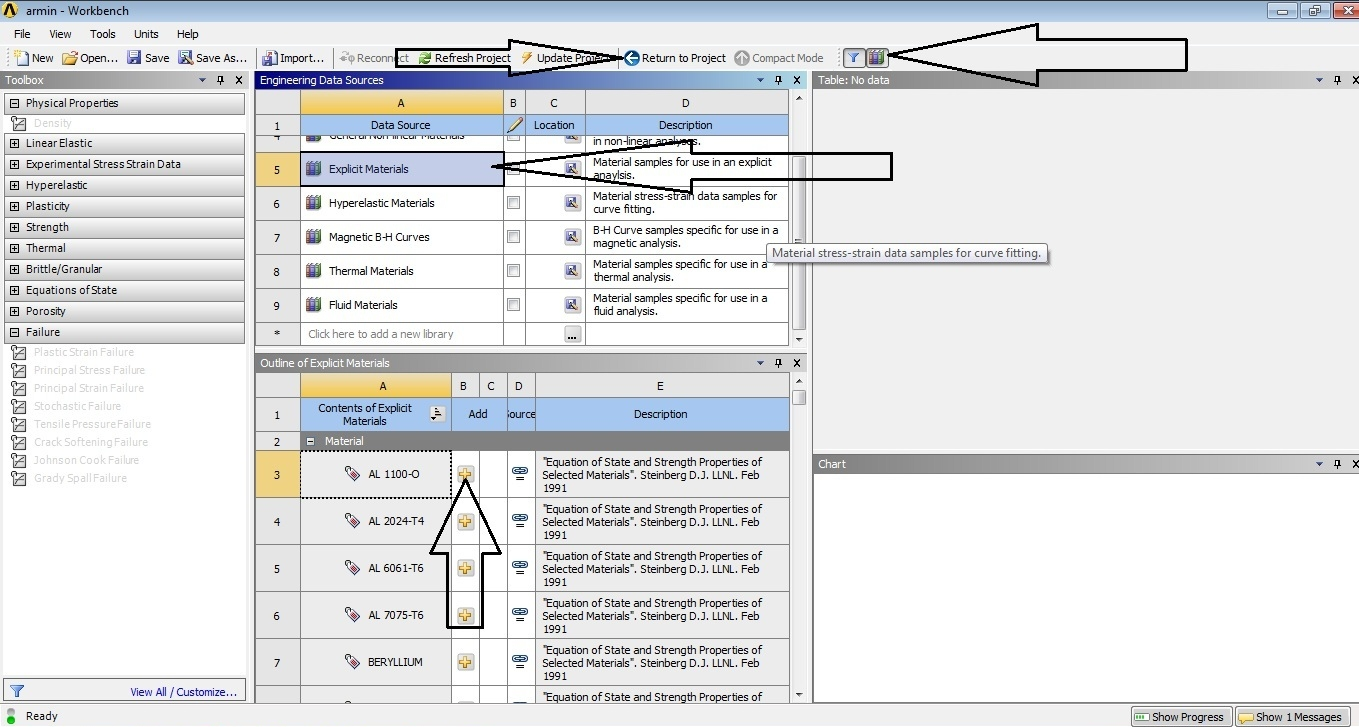9. Step 9: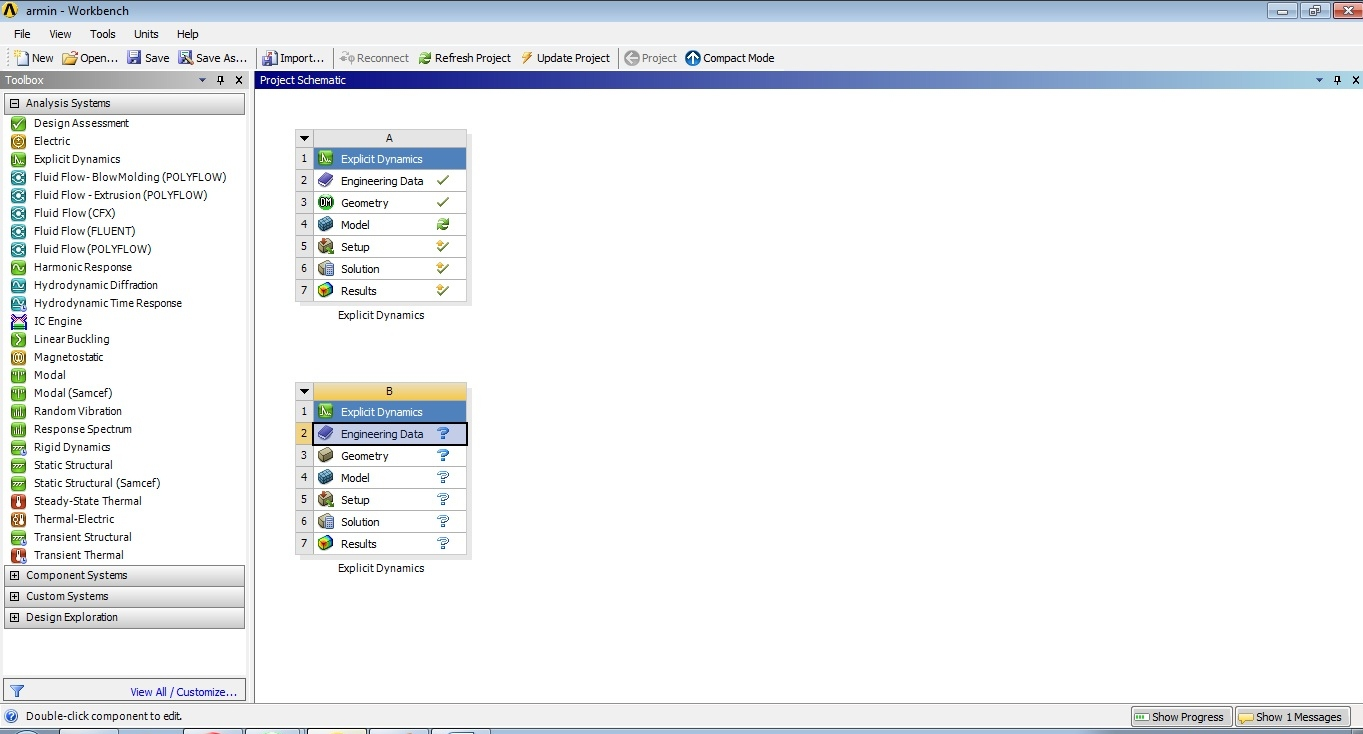10. Step 10: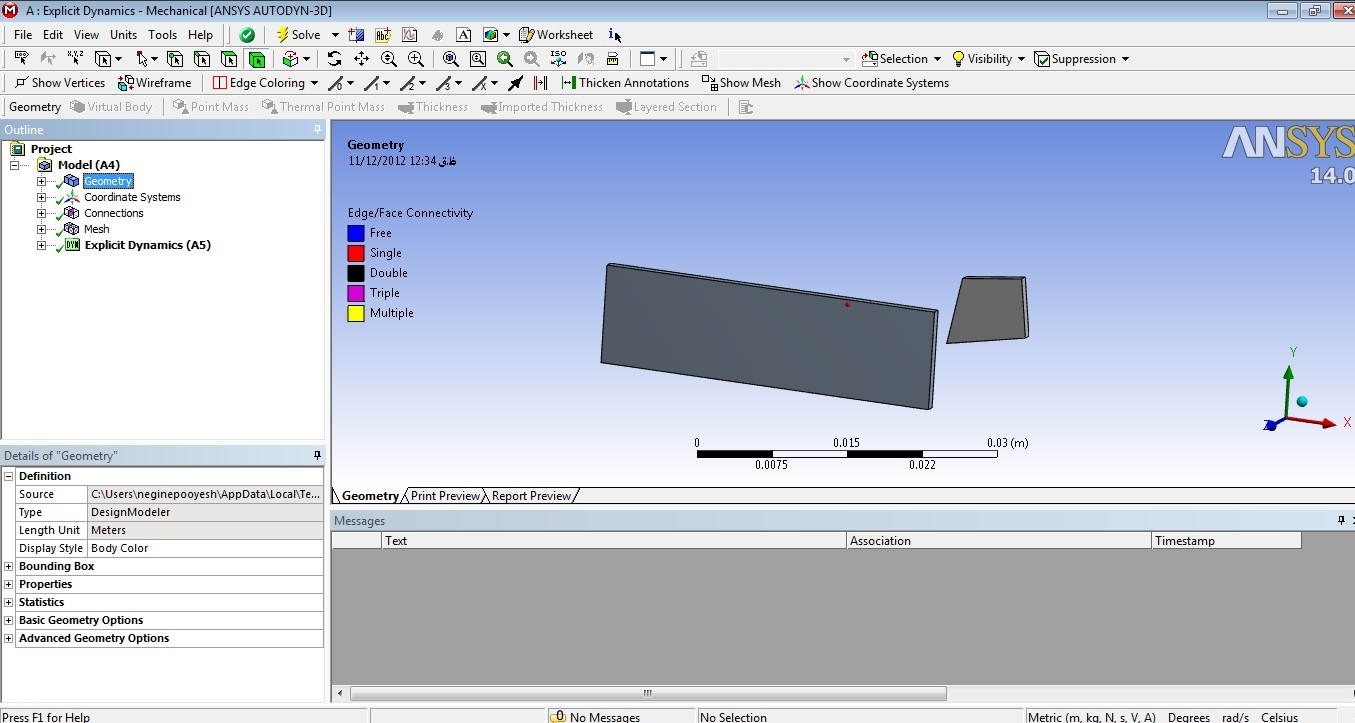11. Step 11:12. Step 12: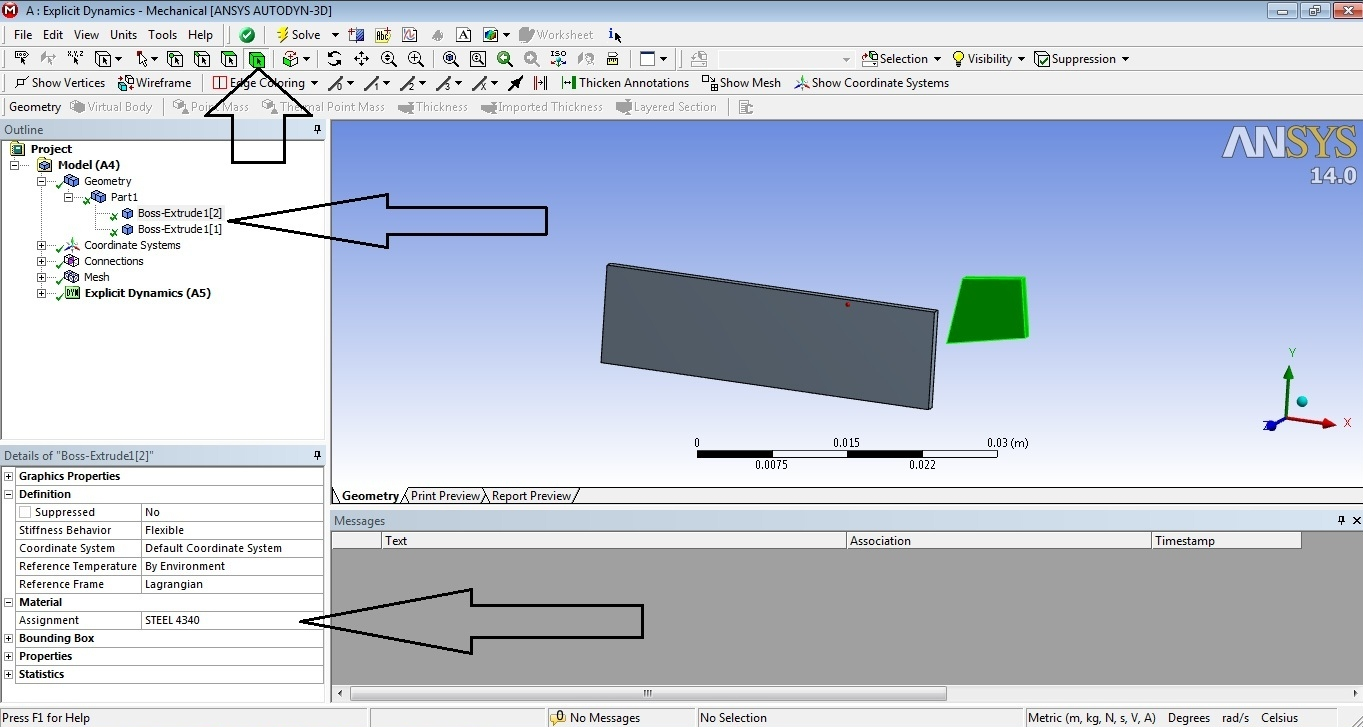13. Step 13: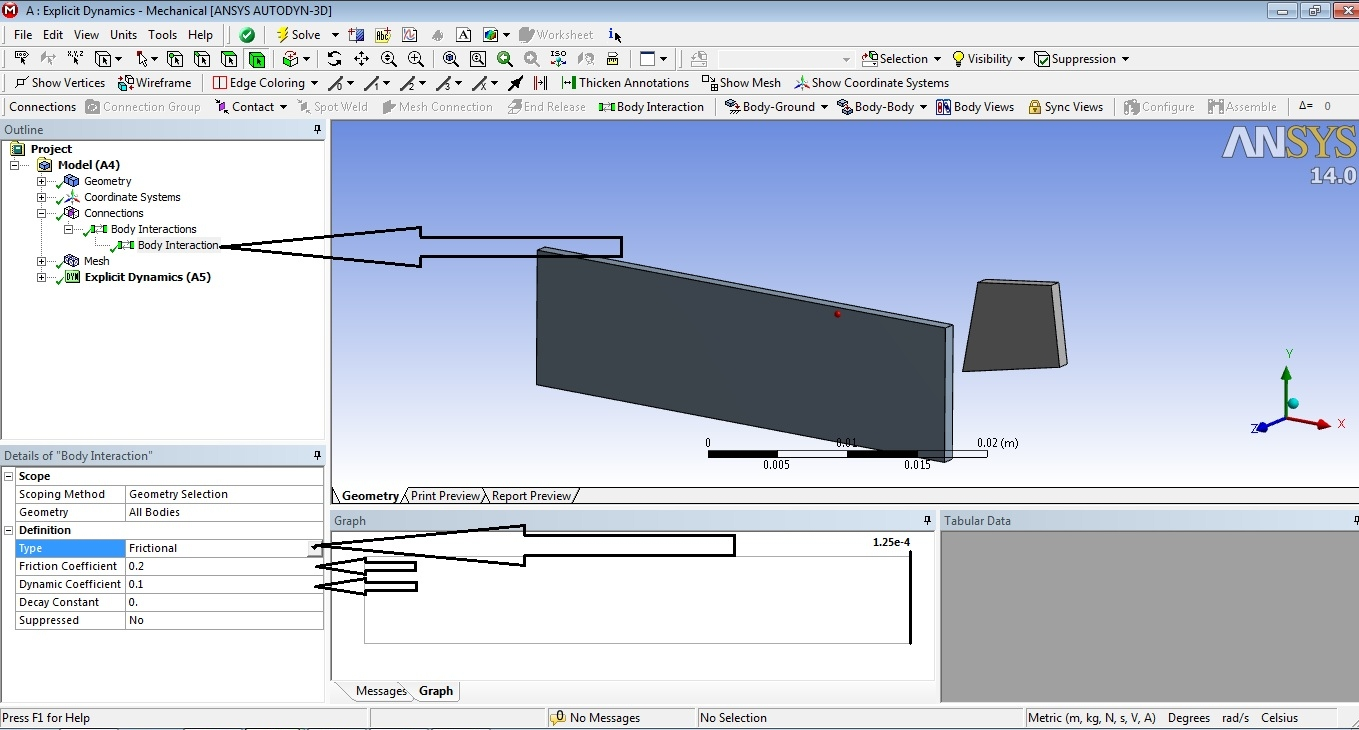14. Step 14: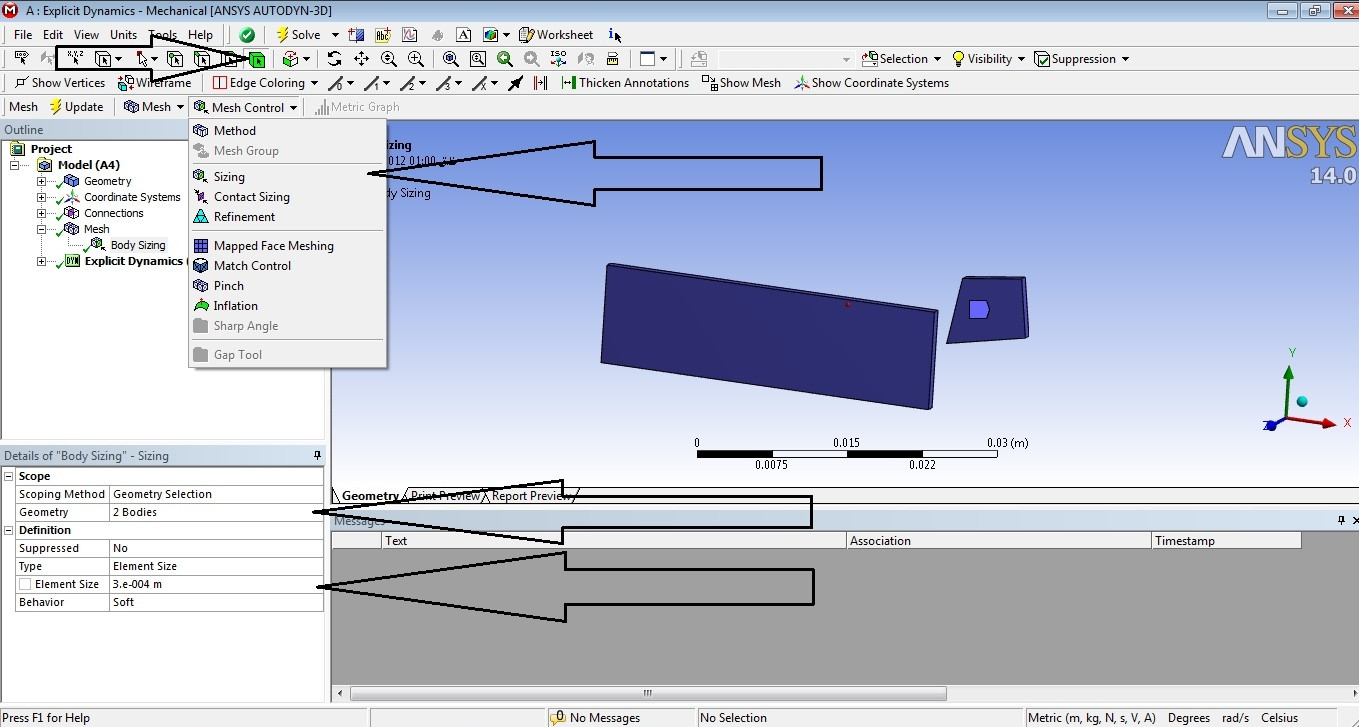15. Step 15: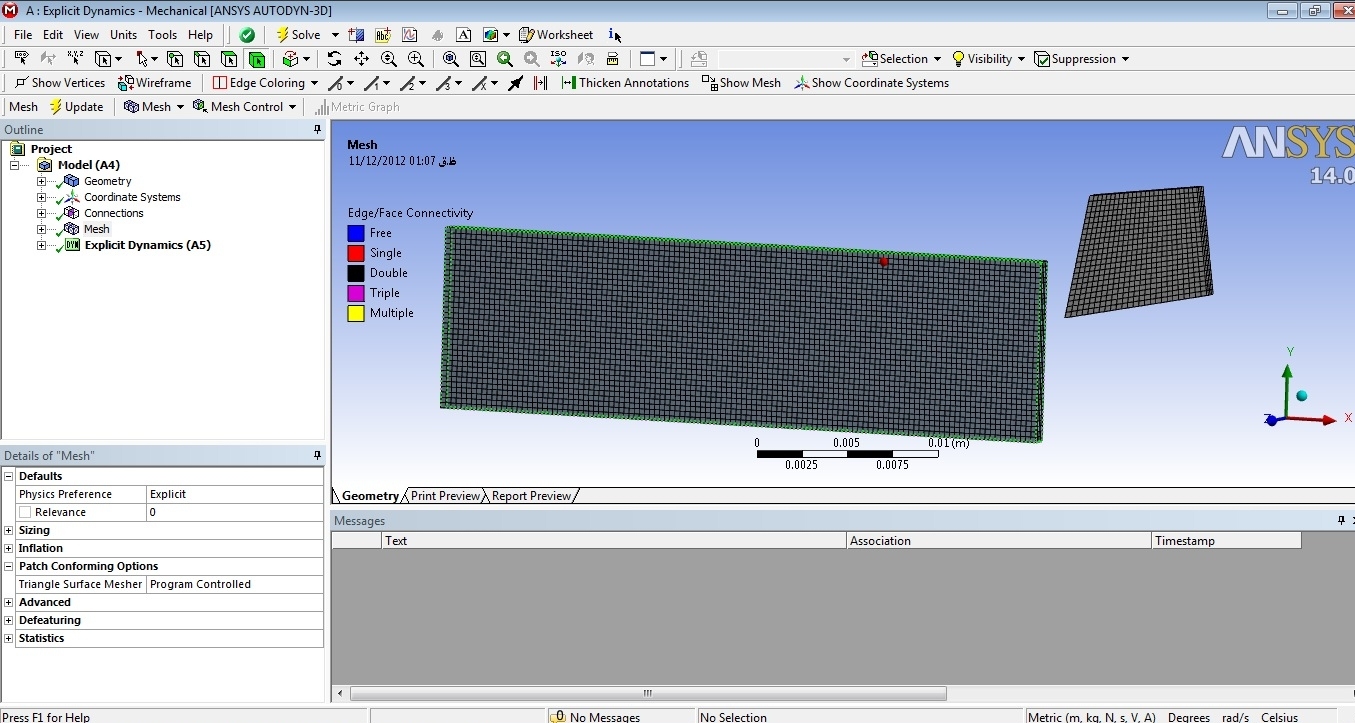16. Step 16: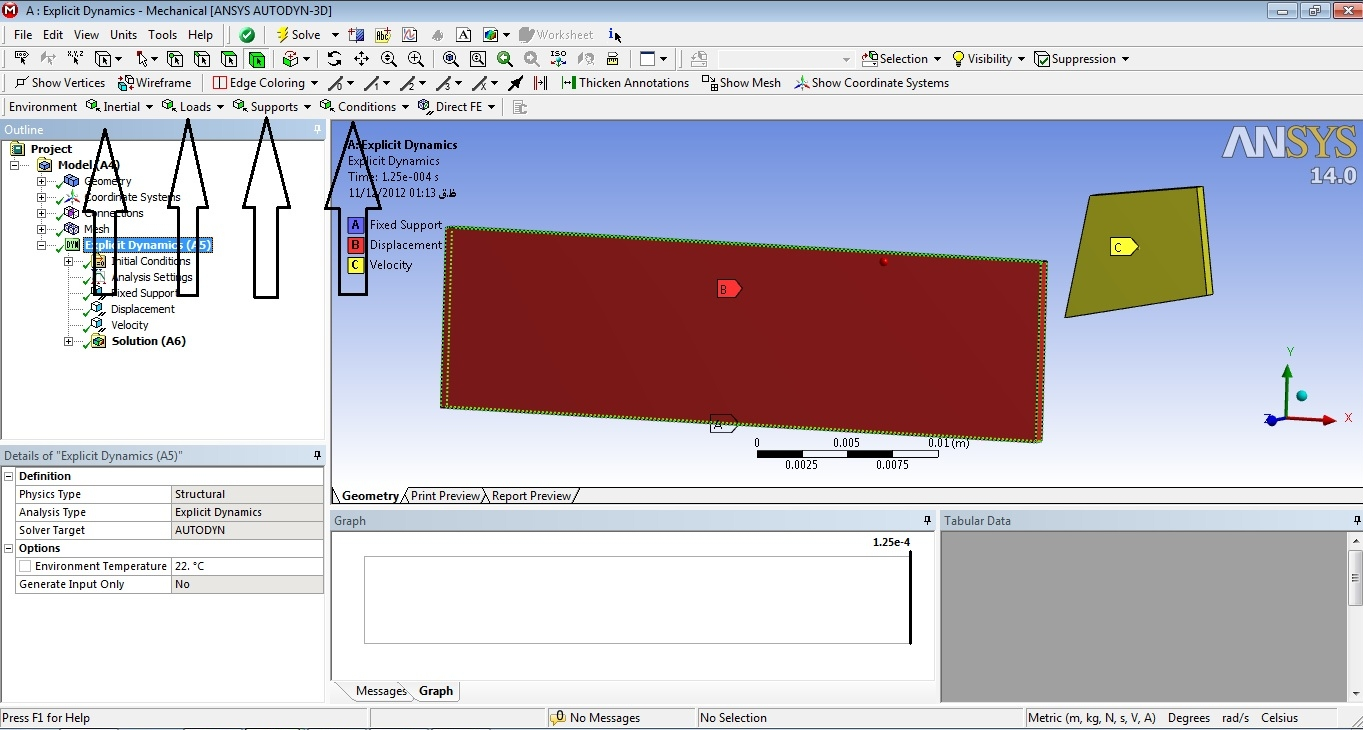17. Step 17: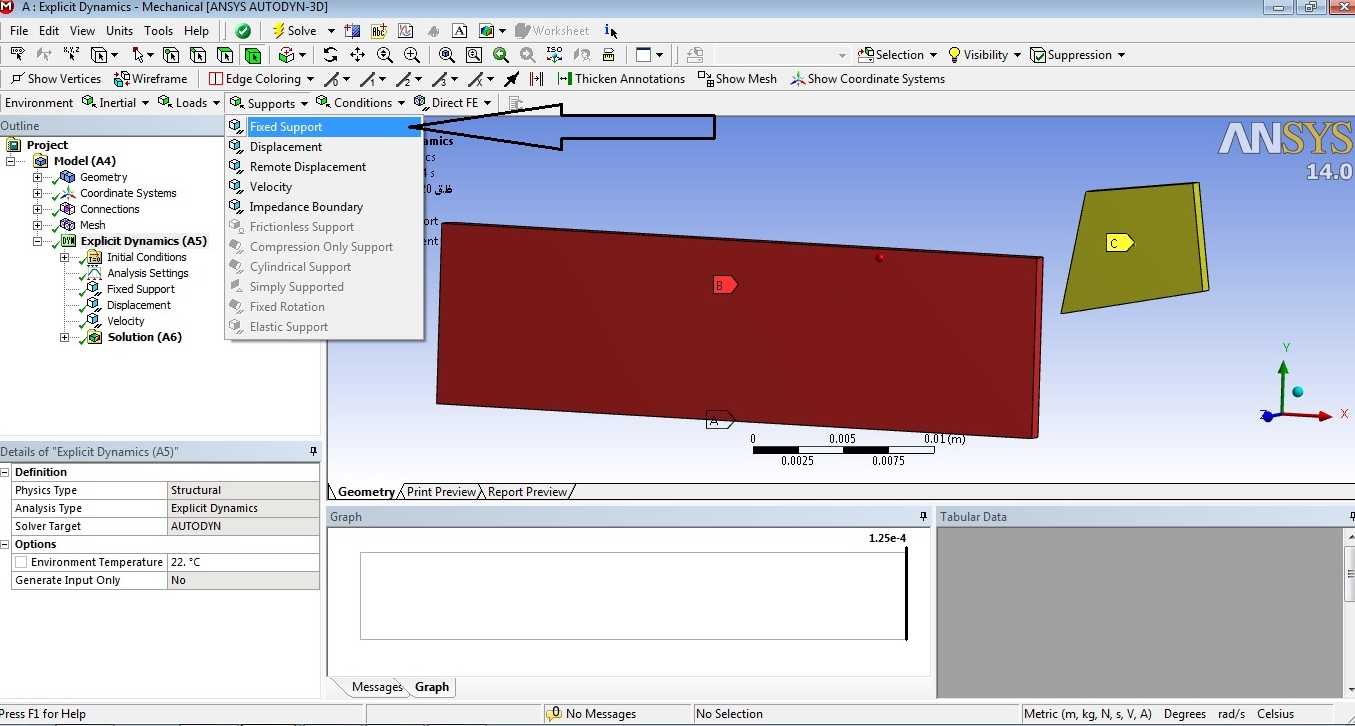18. Step 18: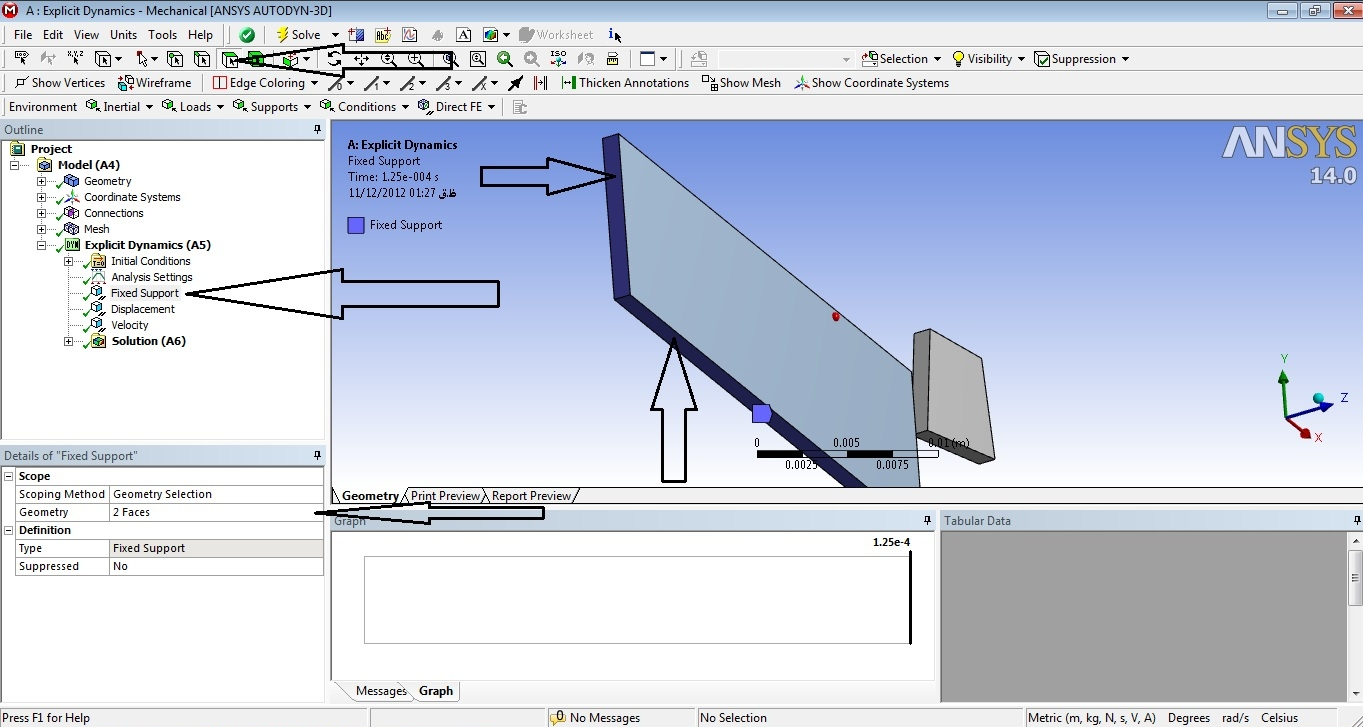19. Step 19: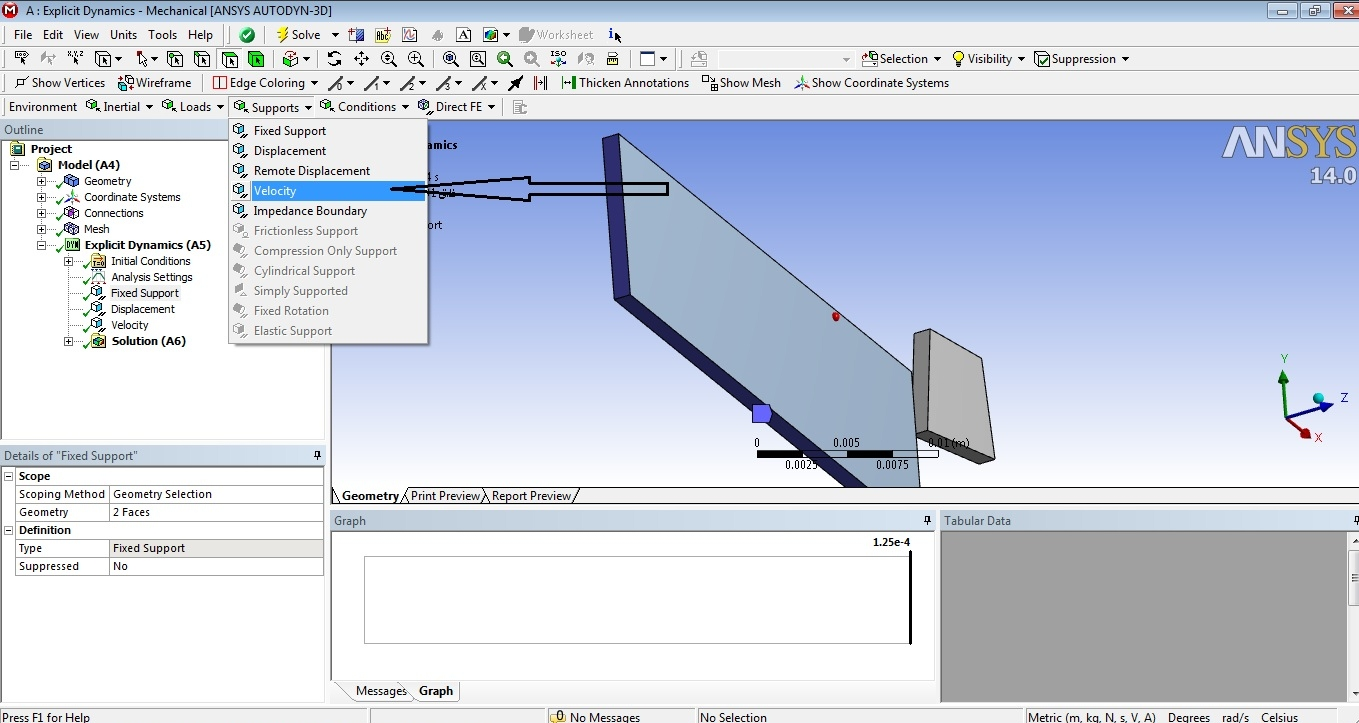20. Step 20: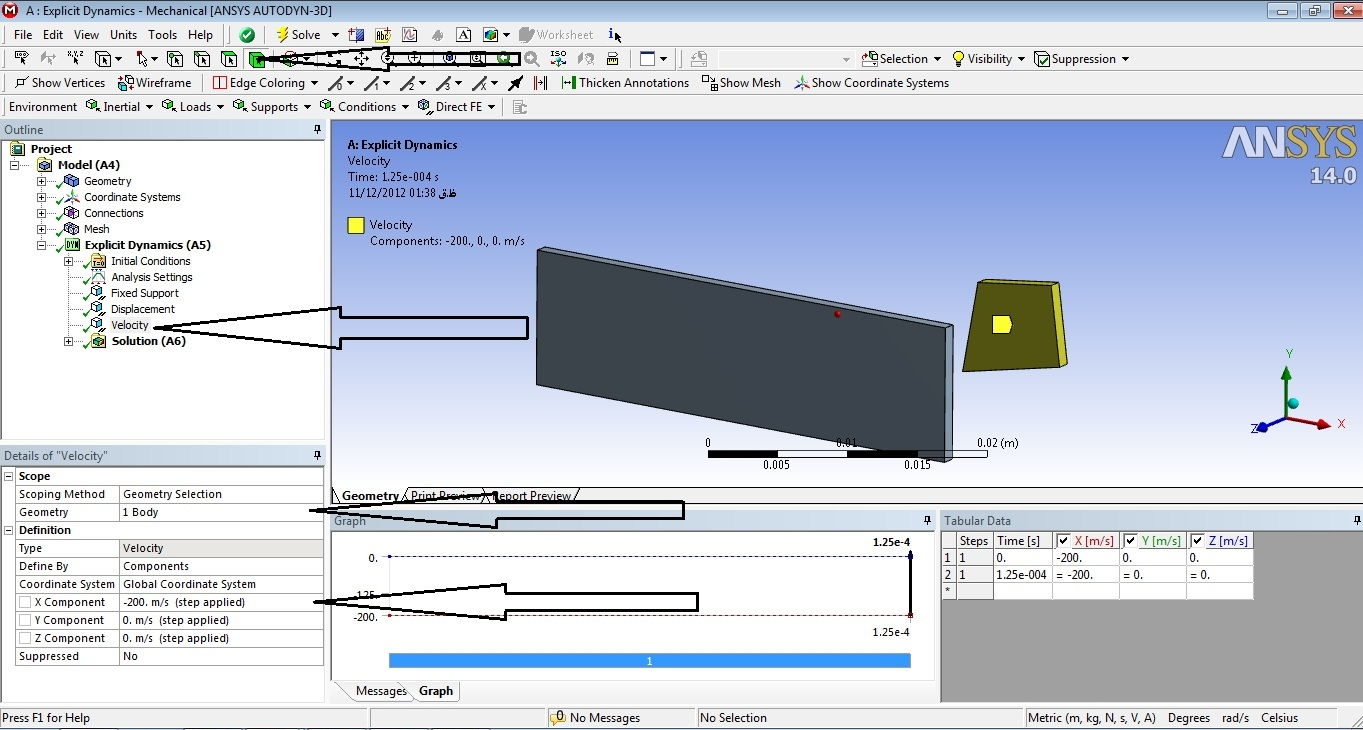21. Step 21: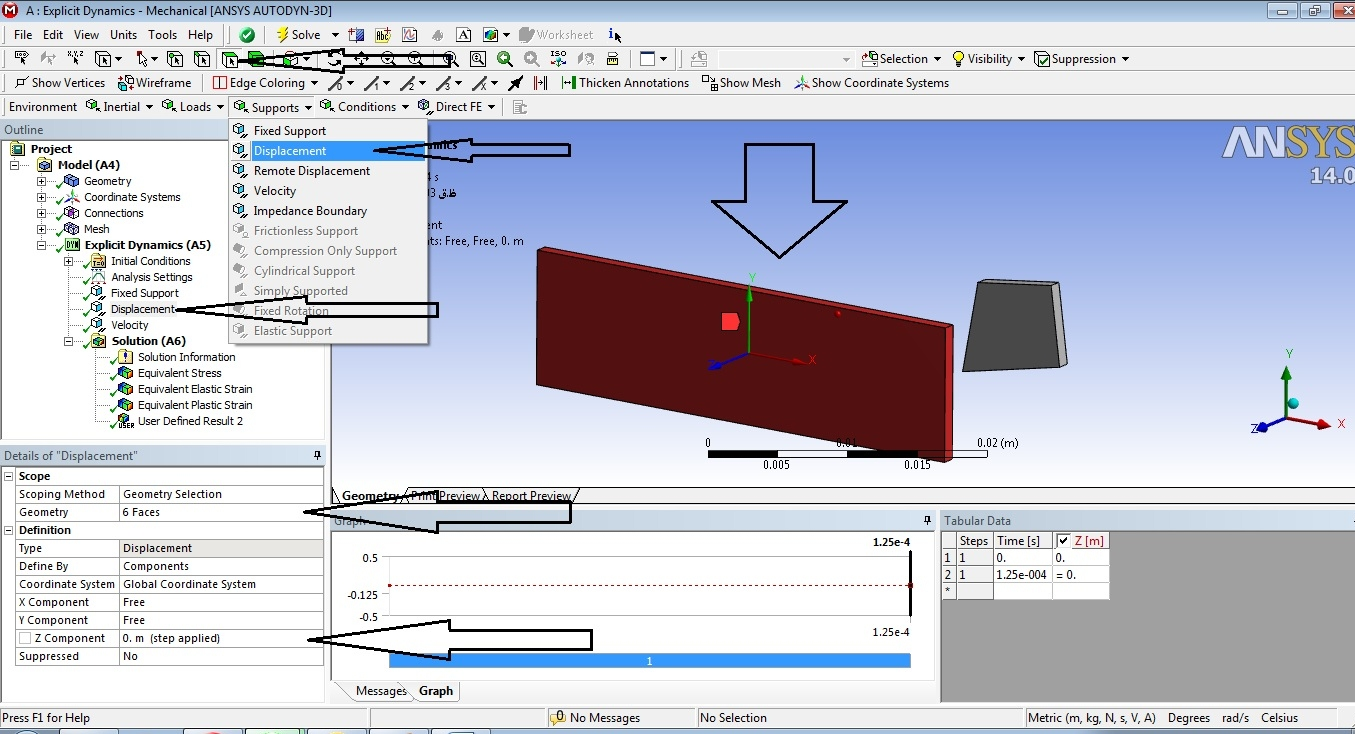22. Step 22: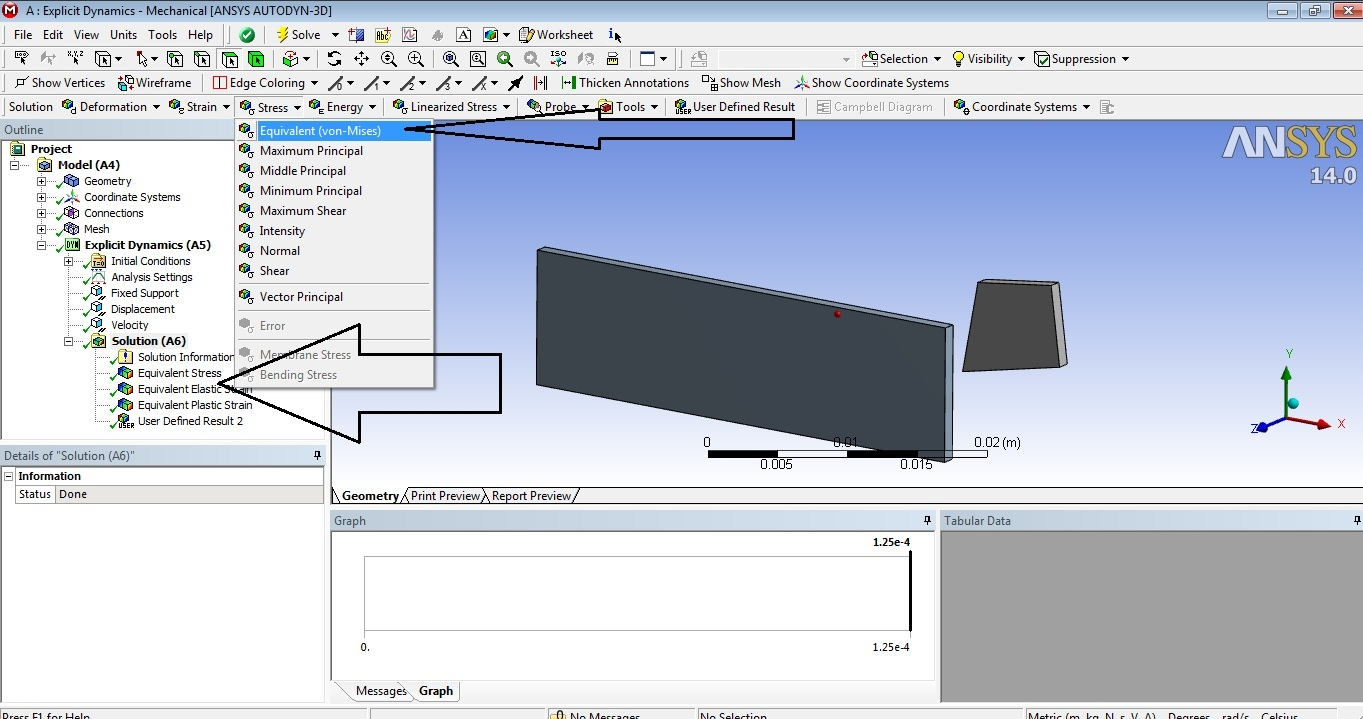23. Step 23: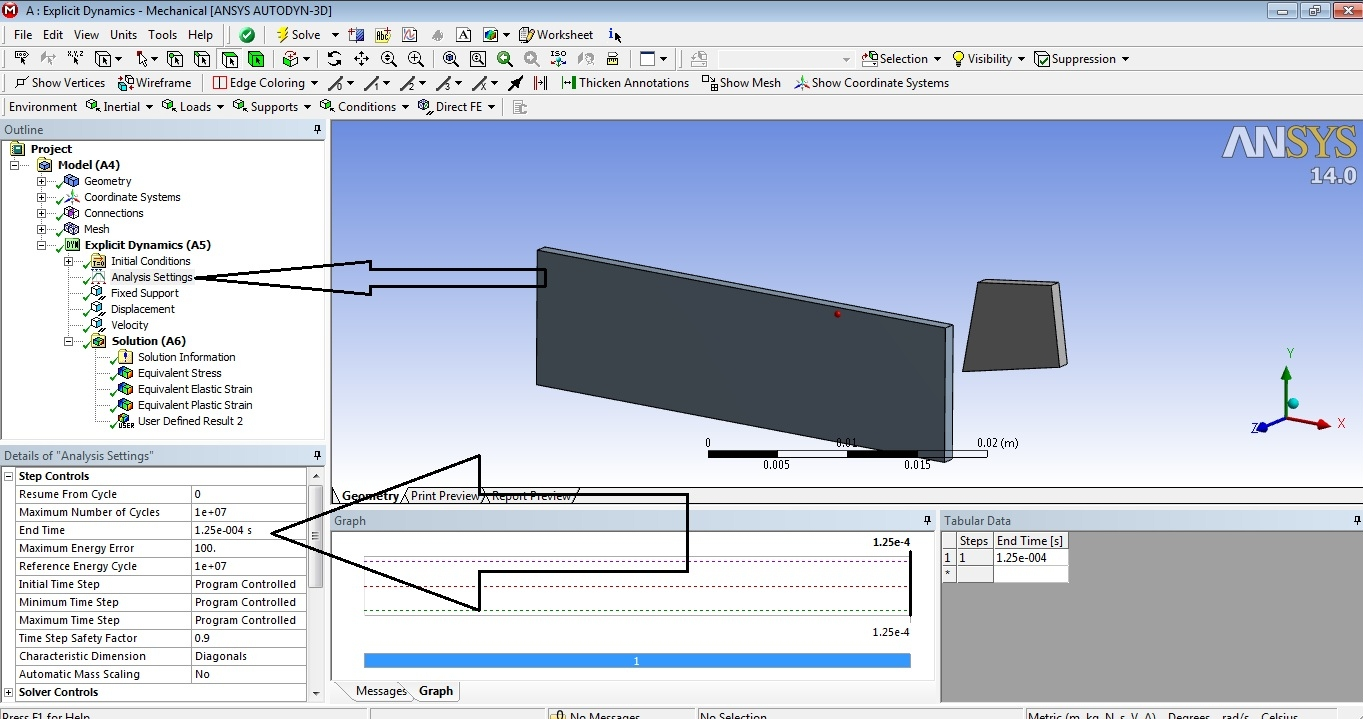24. Step 24: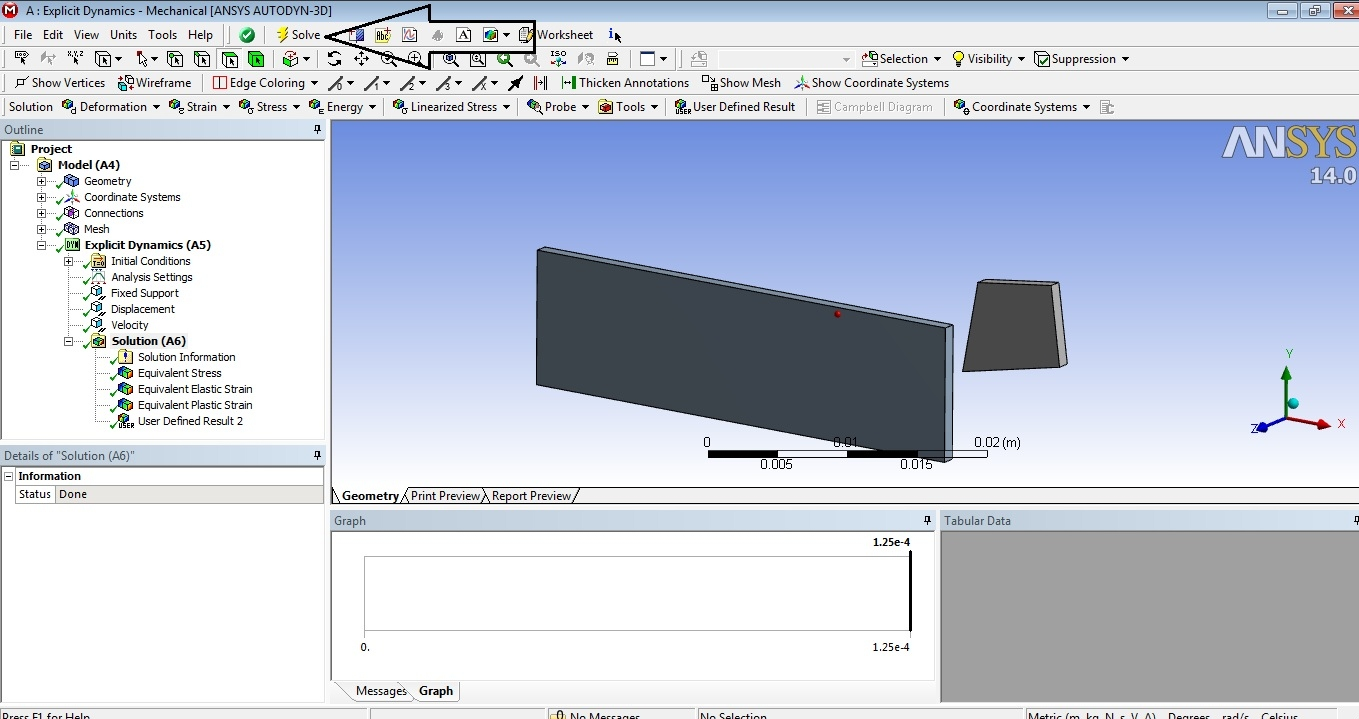25. Step 25: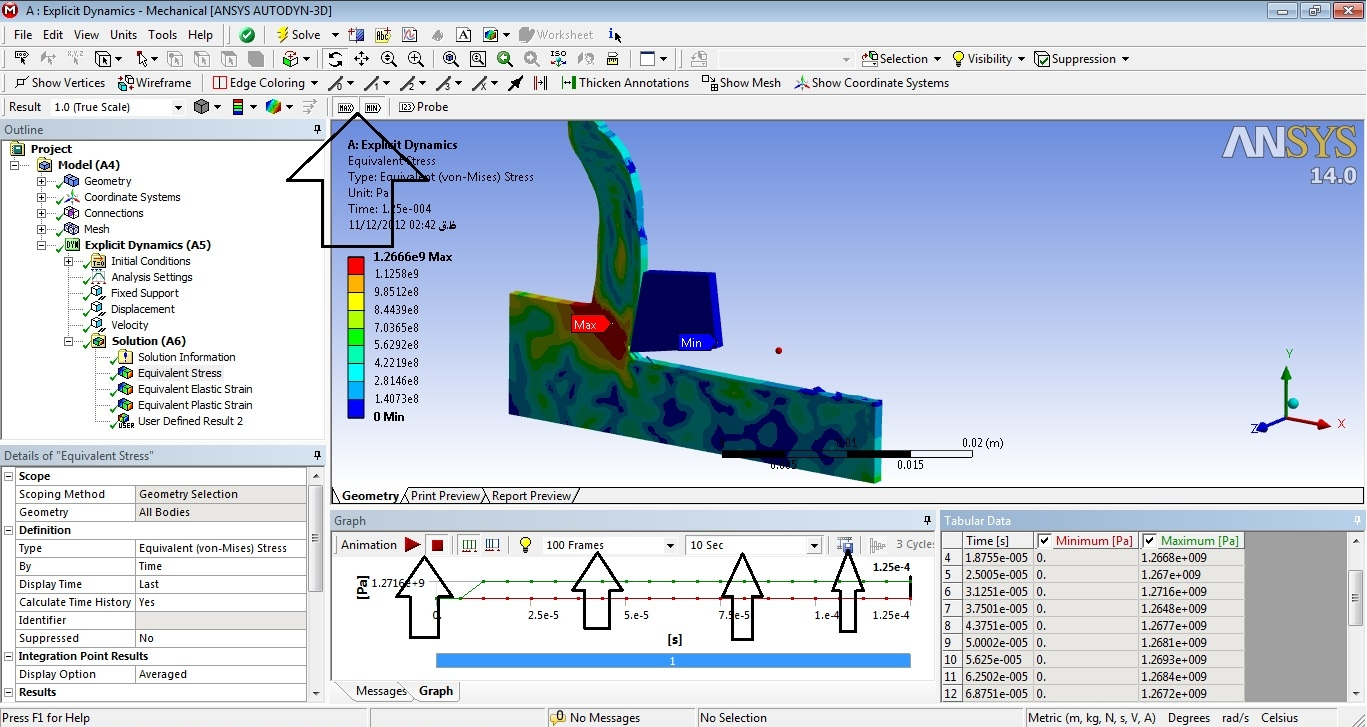26. Step 26: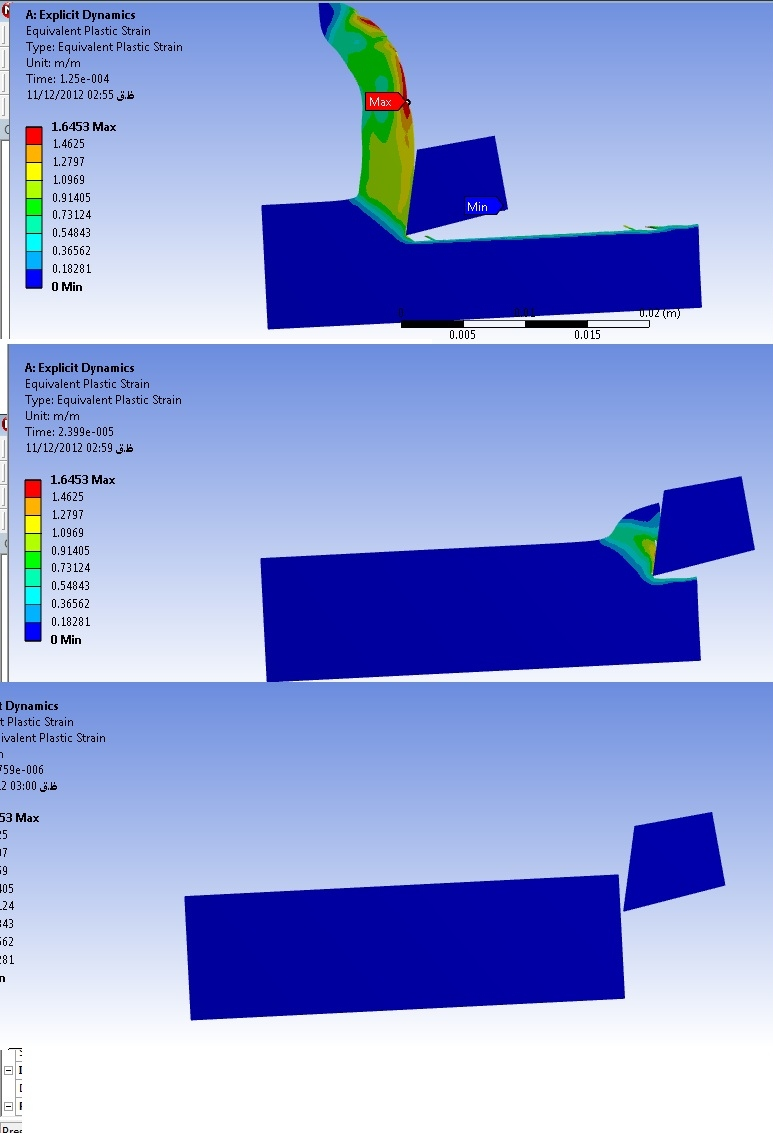27. Step 27: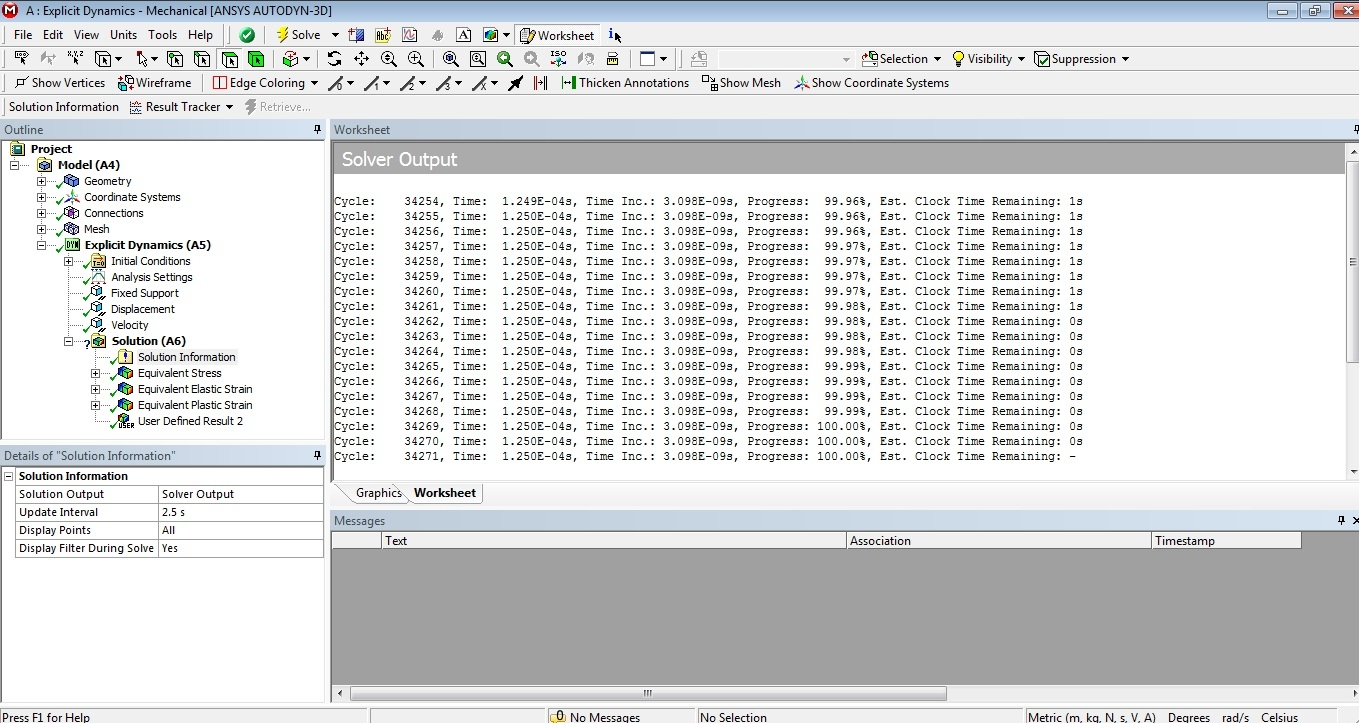28. Step 28: